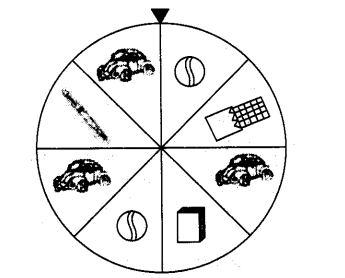# At a birthday party,

Question:

At a birthday party, the children spin a wheel to get a gift.Find the probability of

(a) getting a ball

(b) getting a toy car

(c) getting any toy except a chocolate.

Solution:

(a) The probability of getting a ball

$=\frac{\text { Number of events of getting a ball }}{\text { Total number of events }}=\frac{2}{8}=\frac{1}{4}$

(b) The probability of getting a toy car

$=\frac{\text { Number of events of getting a toy car }}{\text { Total number of events }}=\frac{3}{8}$

(c) The probability of getting any gift except a chocolate

$=\frac{\text { Number of events of getting any gift except a chocolate }}{\text { Total number of events }}=\frac{7}{8}$